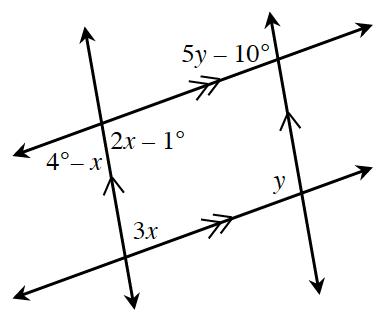### Home > INT2 > Chapter 3 > Lesson 3.2.2 > Problem3-86

3-86.

Multiple Choice: Which equation below is not a correct statement based on the information in the diagram?

1. $3x + y = 180^\circ$

1. $2x-1^\circ=4^\circ-x$

1. $2x-1^\circ=5y-10^\circ$

1. $2x-1^\circ+3x=180^\circ$

B because $2x-1^\circ$ and $4^\circ − x$ are a linear pair so $2x − 1^\circ + 4^\circ − x = 180^\circ$.